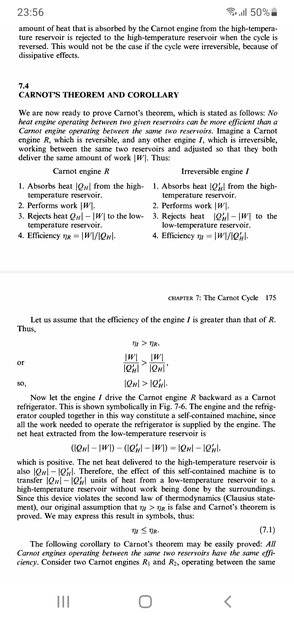# Disproving Carnot's Theorem: Is There an Error in My Thinking?

• I
• phymath7

#### phymath7

TL;DR Summary
I was going through the proof of carnot theorem given in the book 'Heat and thermodynamics ' by Zemansky(seventh edition ,page 174).Here the proof was conducted by considering that the efficiency of the carnot engine is less than the other arbitrary engine.The other engine is driving the reverse carnot refrigerator .That leads to a contradiction hence carnot's theorem is proved.
But if I would assume that the efficiency of the carnot's engine is greater than the other engine and the carnot engine is driving the other engine backward as a refrigerator ,that would lead to the same contradiction hence disproving carbot's theorem! Is there something wrong I have done? Perhaps.Please point me out.•